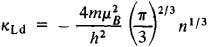# Landau Diamagnetism

The following article is from The Great Soviet Encyclopedia (1979). It might be outdated or ideologically biased.

## Landau Diamagnetism

the diamagnetism of free electrons in an external magnetic field; discovered by L. D. Landau in 1930. The magnetic properties of an electron gas placed in a magnetic field H result from electrons having intrinsic spin magnetic moment and from the change in the character of the motion of free electrons under the influence of the field H. The magnetic field bends the trajectory of the translational motion of the electrons in such a way that the projection of their motion on a plane perpendicular to H assumes the form of closed trajectories (orbits). The resultant quasiperiodic motion of the electrons in an orbit is quantized and gives the diamagnetic contribution кLD to the magnetic susceptibility of the electron gas; the spin moment of the electrons is responsible for the paramagnetic part of the susceptibility. Calculation shows thatwhere n is the density of the electron gas, m is the mass of an electron, μβ is the Bohr magneton, and h is Planck’s constant. In absolute value, the paramagnetism of free electrons exceeds the Landau diamagnetism by a factor of 3. In ordinary measurements of the magnetic susceptibility of paramagnetic metals, the algebraic sum of the diamagnetic and paramagnetic susceptibility of both the electron gas and the ions of the crystal lattice is actually determined. By using the methods of electron paramagnetic resonance, however, it is possible to determine the paramagnetic component alone, and, consequently, the diamagnetic component as well.

At low temperatures, the magnetic susceptibility of metals (diamagnetic and paramagnetic) shows an oscillating dependence on the magnetic field H.

### REFERENCE

Vonsovskii, S. V. Magnetizm. Moscow, 1971.

L. P. PITAEVSKII